# What lies beneath Public-Key-Cryptography?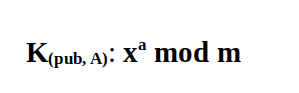Alice performs this operation with her corresponding private key a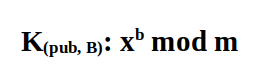Bob performs this operation with his corresponding private key b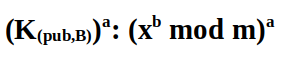The key Alice received from Bob and performs exponentiation with her private key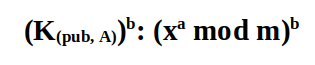The key Bob received from Alice and performs exponentiation with his private key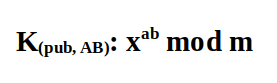This is the agreed upon public key which is same for both parties even while keeping their inputs secret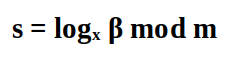Given x and m, compute the logarithm of β base x mod m

# Some important Caveats and points:

• While the above was tried with simpler numbers, the actual numbers are chosen based on a certain criteria. For instance, the integer factorization problem becomes hard for numbers that have a large number of digits and specifically where n (the number to be factored) is a product of prime numbers.
• The Diffie-Hellman parameters are chosen in such a manner that the Discrete Logarithm problem is hard in a chosen integer group particularly cyclic groups of prime order (The discussion of groups is omitted to keep this as simpler as possible and limited to numbers only).
• RSA has been dropped from TLS 1.3 as the key-exchange mechanism² and uses Ephemeral DH instead.
• The fundamental key agreement technique Diffie-Hellman is implemented in many technologies like SSH(Secure Shell), IPSec and TLS.

--

--